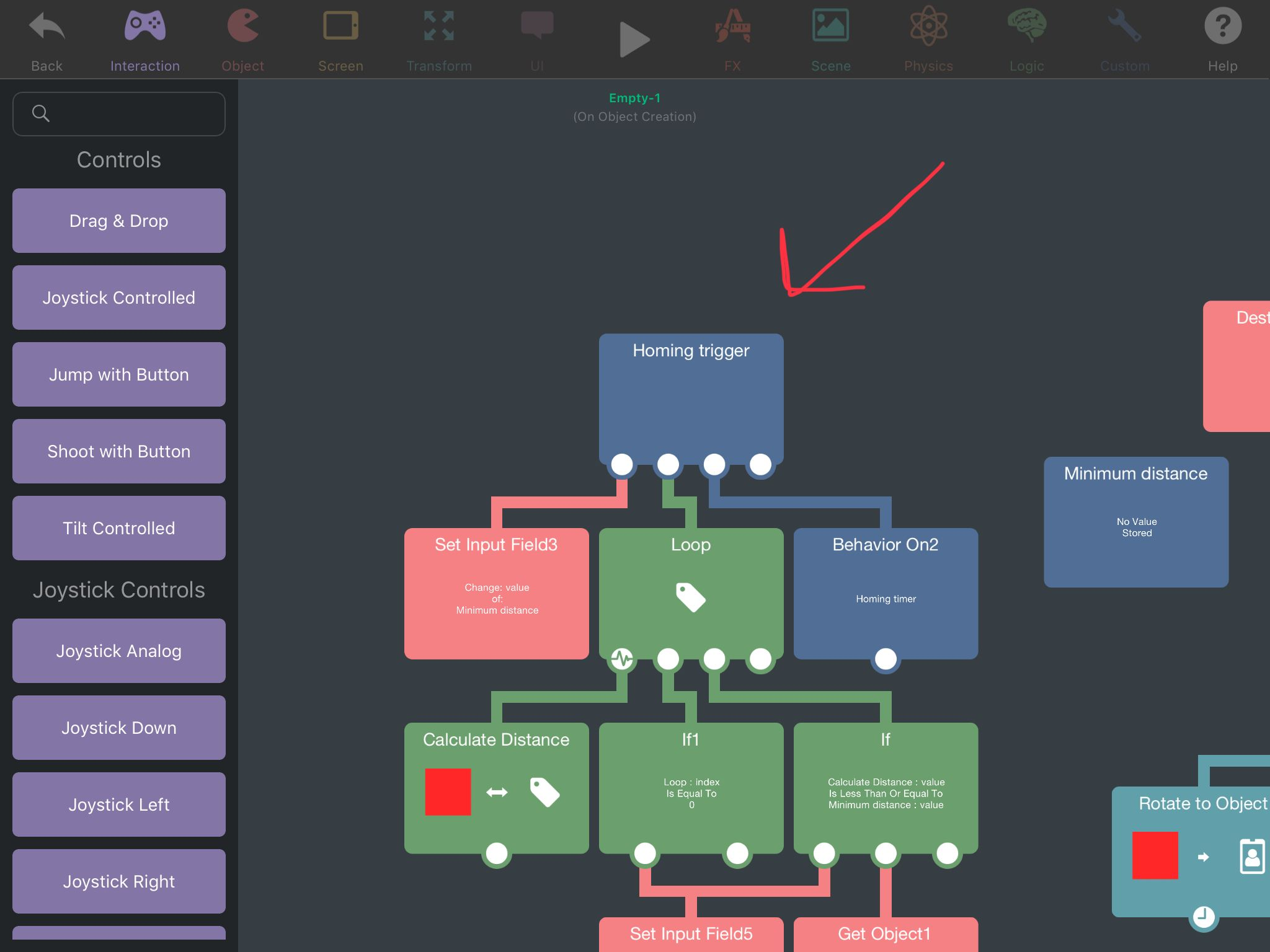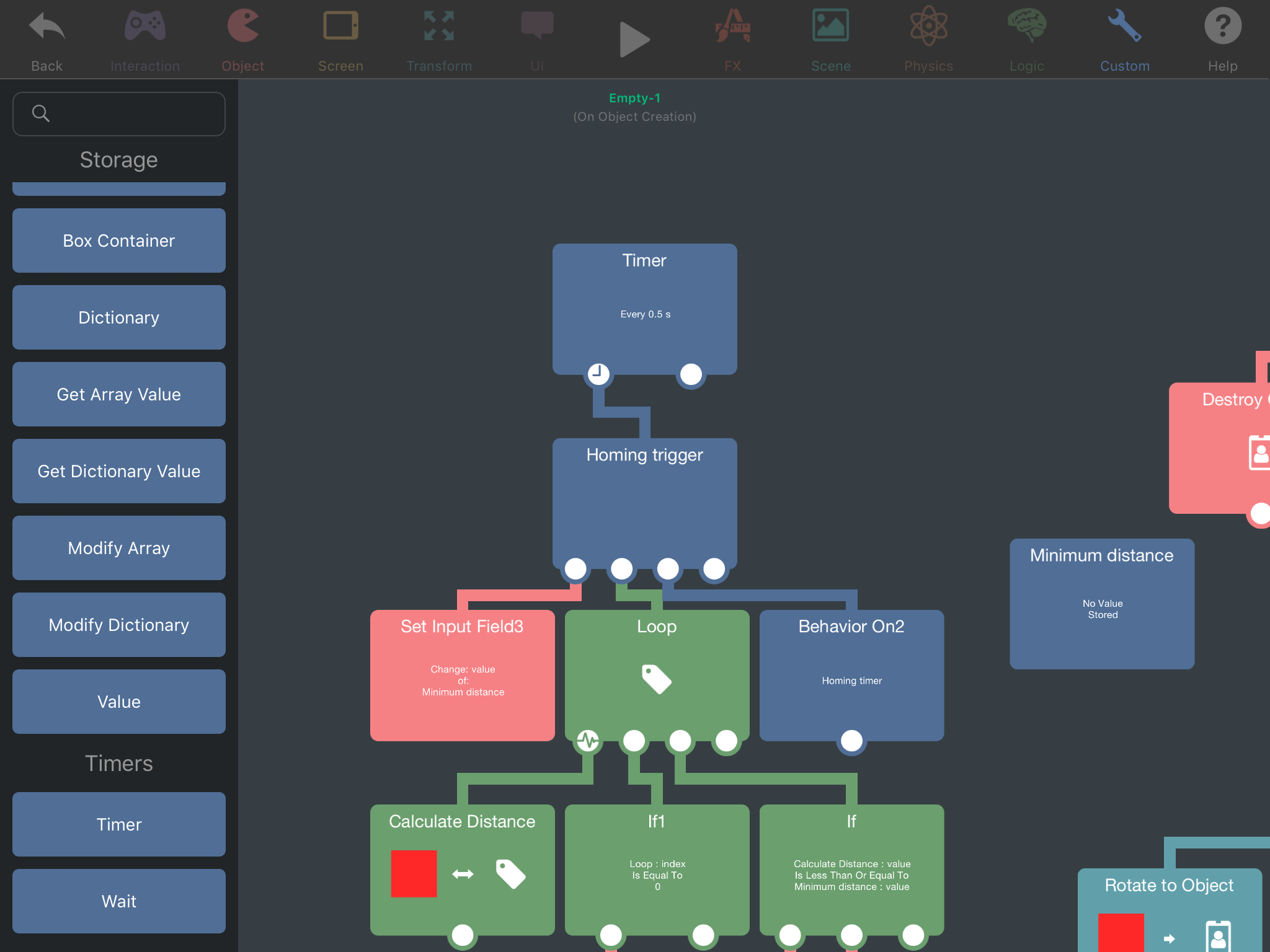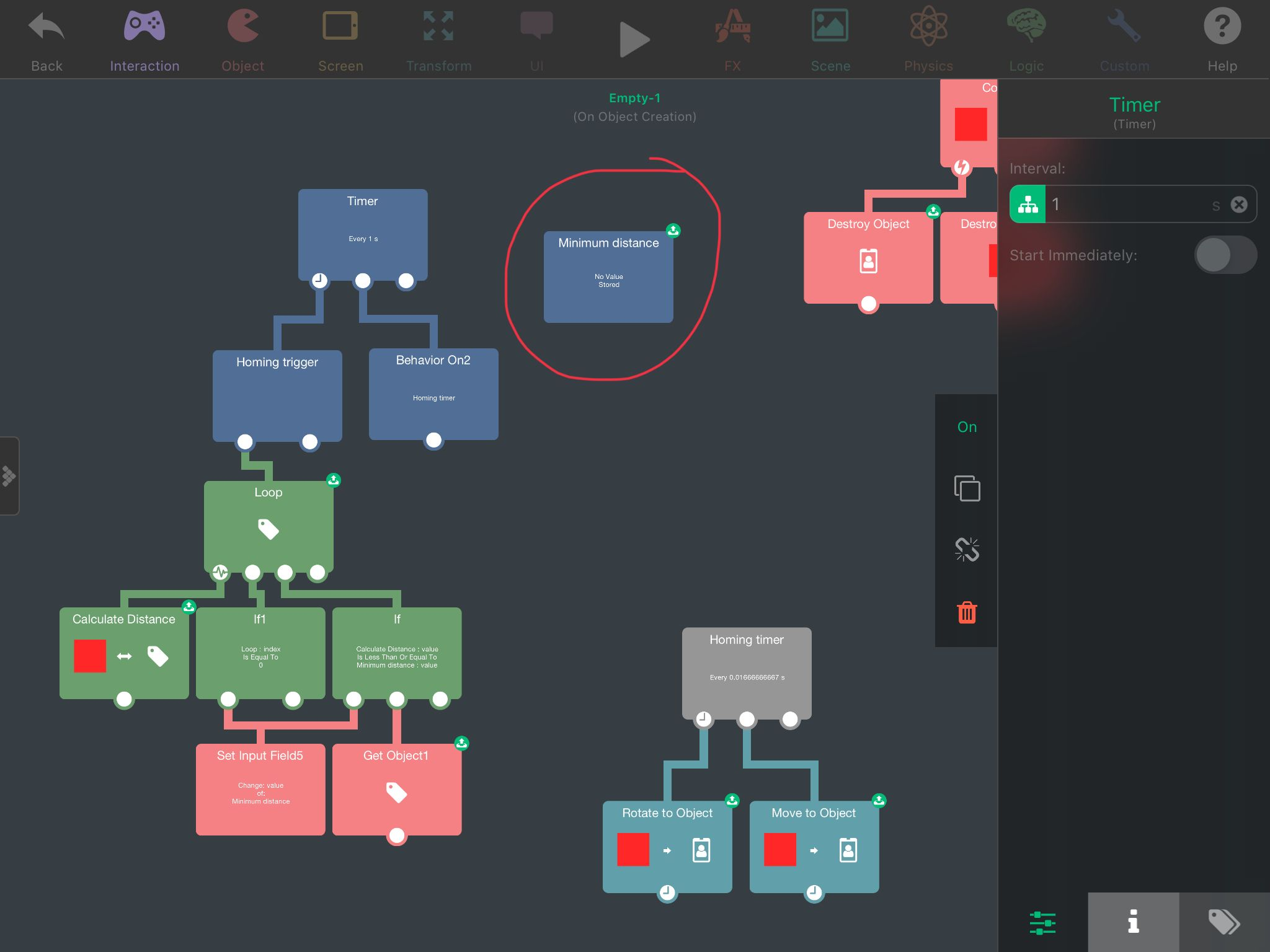Homing projectile vs multiple targets

• @SplitMindGaming If you activate this behaviour in any way (by connecting it under another behaviour directly, using Behaviour On with it selected, or using Execute behaviour with it selected) the missile will redirect targets ONCE.If I do this, the missile will redirect to the nearest target every 0.5s:• @Aidan-Oxley ahhh I didn’t add the timer. Yes it works flawlessly like that. Thanks

• @Aidan-Oxley care to be a teacher for a bit. I don’t understand input field or even how to use loop properly in that matter. What does it mean when you put “a” on the input field. What does that represent?

• @SplitMindGaming lol nothing. It's a mistake, I meant to put 0 or absolutely nothing in there. Now that I think about it, delete that behaviour completely, it'll still work exactly the same, it's leftover from my previous method before Jack showed me better one.

Set Input Field basically does exactly as it says. When you press on that symbol next to the Input field of a behaviour, you are given a prompt and you can create a Set Input Field behaviour. When this behaviour is run, it simply changes whatever was in there to the new value you put in it.

The loop behaviour is like a timer but extremely fast, as in it will freeze hyperPad until it is done (it won't freeze hyperPad unless you make it loop a crazy amount of times). It has a few settings, you can either set the exact amount of times you want it to repeat the stuf under it, or you can give it a tag and set it to "for each" where it will count the amount of objects in that tag by itself, and then repeat the stuff under it once for each object while also selecting that object to do stuff to.

• @Aidan-Oxley I see what about the box container minimum distance. It doesn’t even have any value storing. I notice it was called on an if statement saying if calculate distance is less then minimum distance. How does that calculate when the minimum distance contains no value. Or does it contain a value? I’m not sure exactly what happening there.

• @SplitMindGaming If the loop index = 0 (that is, if it's the very first object selected in the loop), set the minimum distance to the distance calculated to this specific object. Now, if the distance calculated is less than or equal to the value stored in minimum distance, then get the ID of this object we calculated the distance to.

This is exactly how the logic under the loop works in the example, explained into words.

• I’ve gotta say.. thanks a million. I’ve NEVER seen the loop and get object behaviors there. I’ve always wished for them lol.

I got it to work almost perfectly.

Also, I find that using a Propel Object behavior with a negative value attached to a timer is a nice, smooth, and easy way to accomplish a classic homing missile effect.

• @bosswave Yeah I've used that before I think. Just as long as you have friction it's fine (without friction the missile can just go into orbit of the target lol).

• @Aidan-Oxley Think you mean air resistance rather than friction :P

• @Jack8680 Shush same thing. Air resistance your face.

• @Aidan-Oxley couldn’t you just put the actual number 0 on that if statement instead of calling a box container? Or does it not work that way. Also to minimize timers. Could you also set the “rotate to object” and “move to object” directly under the homing trigger?

• Could you also set the “rotate to object” and “move to object” directly under the homing trigger?

You probably could. The thing is, the homing trigger is only meant to select a target, not move the missile to the target. That's what the Homing timer was for. As bosswave did, you can completely change the homing mechanics to however you want as long as you use the same Object ID in your homing logic as the example does.

I'm not exactly sure what you mean with using 0 instead of the box container, but I suspect it doesn't work the way you think?

• @Aidan-Oxley I see. Im still confused on the box container titled “minimum distance”. It says there’s no value being stored. So how is it calculating the minimum distance? Sorry it might be really obvious to you but I can’t seem to wrap my head around what is going on here.• @SplitMindGaming The value is set by the Set Input Field5 behaviour

If calculate distance is less than minimum distance, set minimum distance to calculate distance.

• @Jack8680 ohhh ok that’s where that input field came from. I got distracted from the other input field that had an “a” on it

• Ok one last question. Sorry Bosswave I know this is your post and I’m kind of taking over it lol. So on the if statement you put if loop Index is = to 0. 0 represents the first item on the list of loops correct. So if I put a 1. What will happen?

• Actually the more I look at it the more I get confused. I’m not understanding how this small piece of logic is doing all these things. Ok so in idiot terms. Minimum distance is a set input field. On that set input field you place the “calculate distance” ok makes sense. But on the other if statement it has if calculate distance is less the minimum distance. But aren’t you putting the calculate distance within the minimum distance because your using the input field to capture the calculate distance. So in reality the if statement is reading the same thing basically which is “ if calculate distance equals calculate distance right. I think I have a migraine now.

• @SplitMindGaming If you put 1 in the If statement that is looking at the loop index, then it will apply the logic to the second object it looks at. I think the order of the loop goes from oldest spawned object up to newest. If I'm right about this, then setting it to 1 will mean the oldest spawned target will always be completely ignored by the homing missiles.

• @Aidan-Oxley lol you don’t need to answer my last question. Honestly I still won’t probably get it. You would have to really dumb it down for me by using apples and bananas as a metaphors at this point because that was all too confusing for me.

• Let me try to give you an example, for example there are 3 targets in the world. Let's call them object a b and c, where a is the oldest one and c is the youngest one (the last one spawned). Object a is a medium distance away, it's sitting 5m away from the missile. Object c is 7m away from the missile, and object b is right next to the missile being 2m away. Now the objects are in these places, let's say the homing logic is triggered right now, with the objects in these places:

The loop has target as its tag, and it sees 3 targets (hyperPad does this automatically), so the loop is going to trigger all the behaviours underneath it 3 times. The first time is run, and the loop index is now 0. It calculates the distance to object a (the oldest) first, and gets 5. Then it runs the If statement, is index equal to 0? Yes. So now set the Minimum Distance box container to 5. After this is done, it runs the 2nd If statement. Is the calculate distance LESS THAN or EQUAL TO the minimum distance? 5 = 5, so yes. Get the ID of the object we just calculated distance to (which is object a). First loop done.

Second loop, now the index is 1, and it is now looking at object b. Calculate distance, and it gets a value of 2. Does index = 0? No, so the first If statement does nothing. Second If statement, is the calculate distance less than or equal to the minimum distance? The minimum distance is currently still 5 at this point, and 2 is less than 5. So now we set the minimum distance to the calculated distance (which is 2 right now), and get the ID of this object (which is the ID of object b).

3rd and last loop, the index is 2 and looking at object c. Calculate distance, gets 7. Index = 0? Nope, it's 2, do nothing under this If statement. Is calculate distance ≤ minimum distance? 7 is not less than or equal to 2, so do nothing.

The loop is done, now continue on. The homing timer is set to home in on the last object that had it's ID taken, and this is object B, so the missile will now home in on object B, which was the closest one being 2m away.